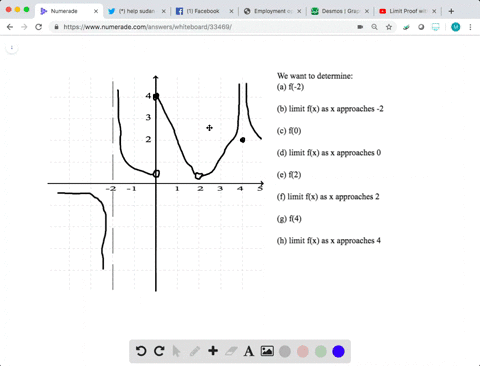Enroll in one of our FREE online STEM bootcamps. Join today and start acing your classes!View Bootcamps### Graphical Reasoning In Exercises 29 and 30 ,use t…

03:39University of California, Berkeley
Problem 29

# Graphical Reasoning In Exercises 29 and 30 ,use the graph of the function $f$ to decide whether the value of the given quantity exists. If it does, find it. If not, explain why.(a) $f(1)$(b) $\lim _{x \rightarrow 1} f(x)$(c ) $f(4)$(d) $\lim _{x \rightarrow 4} f(x)$

## Discussion

You must be signed in to discuss.

## Video Transcript

Okay, so it's important. Ate our function at one. That one. Our function is this black point here. That's equal. Well, let's see the limit as X approaches one from, Oh, this one of our function. So it's sharp working one from the campsite we get, uh, I think Well, for 3.5 and then as your approach one from our right hand side we got one too are not equal for a limited like the person one does not exist. Function applied at four. This is actually this is an opening ball. Here is no valued actor. This is on the line now the limit is X approaches four of our function. Well, when we're approaching from the left hand side were poking too. And then we were When we approach it from the right hand side, we're also looking to so the limited nick with you